1599926400

# Apache Brooklyn- A Step Towards Autonomic Computing

Greetings, fellow developers!!

The control and management of computing systems have evolved from an environment in which a single process running on a computer system to a large, complex and dynamic environment in which multiple processes running on geographically dispersed heterogeneous computers that could span several continents.

## What is Autonomic Computing?

Autonomic computing is a term used by IBM to describe the need to shift the burden of managing IT systems from IT professionals to the systems themselves. Autonomic computing is a computer’s ability to manage itself automatically through adaptive technologies that further computing capabilities and cut down on the time required by computer professionals to resolve system difficulties and other maintenance such as software updates.

#apache #autonomic-computing #open-source #apache-brooklyn #devops

## Buddha Community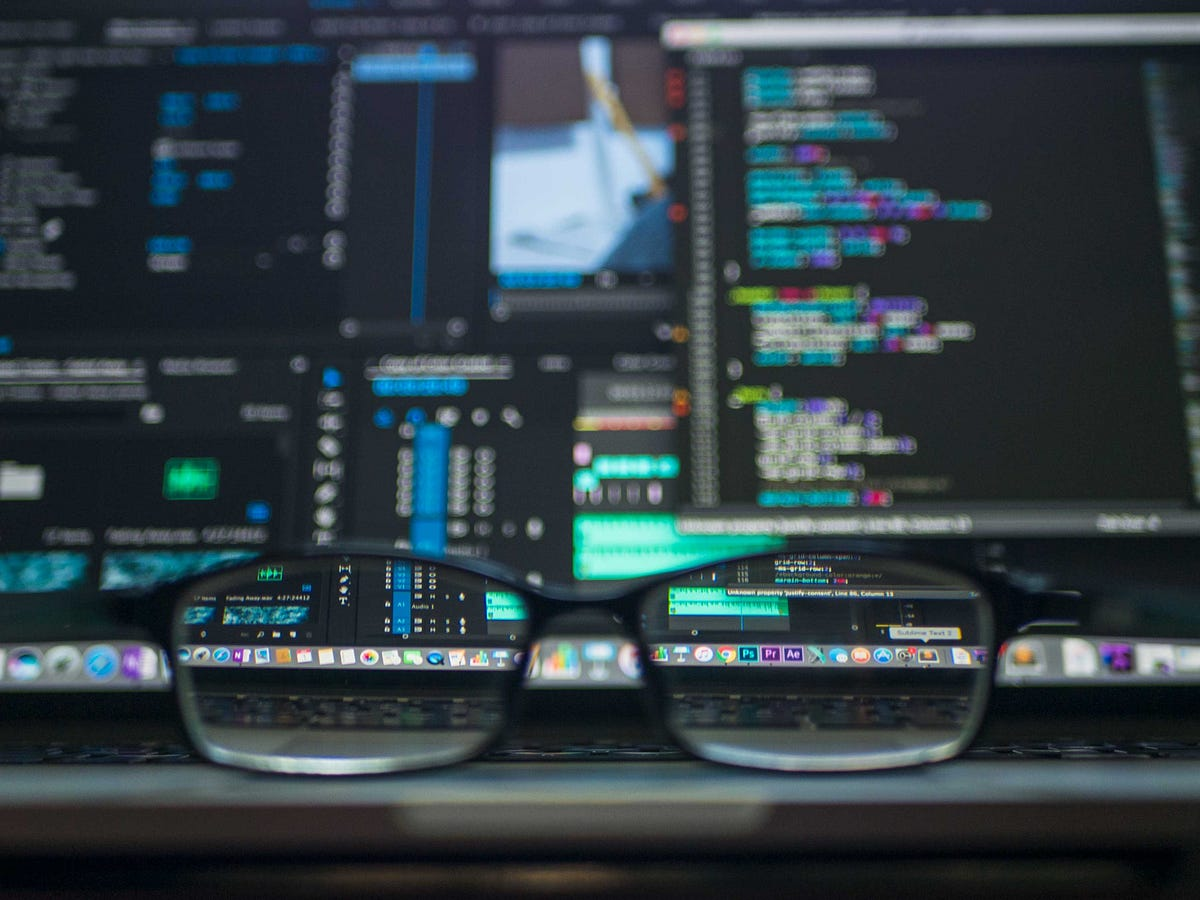1657107416

## Find the Best Restaurant Mobile App Development Company in Abu Dhbai

The era of mobile app development has completely changed the scenario for businesses in regions like Abu Dhabi. Restaurants and food delivery businesses are experiencing huge benefits via smart business applications. The invention and development of the food ordering app have helped all-scale businesses reach new customers and boost sales and profit.

As a result, many business owners are searching for the best restaurant mobile app development company in Abu Dhabi. If you are also searching for the same, this article is helpful for you. It will let you know the step-by-step process to hire the right team of restaurant mobile app developers.

## Step-by-Step Process to Find the Best Restaurant App Development Company

Searching for the top mobile app development company in Abu Dhabi? Don't know the best way to search for professionals? Don't panic! Here is the step-by-step process to hire the best professionals.

#Step 1 – Know the Company's Culture

Knowing the organization's culture is very crucial before finalizing a food ordering app development company in Abu Dhabi. An organization's personality is shaped by its common beliefs, goals, practices, or company culture. So, digging into the company culture reveals the core beliefs of the organization, its objectives, and its development team.

Now, you might be wondering, how will you identify the company's culture? Well, you can take reference from the following sources –

• Social media posts
• App development process
• Client testimonials

#Step 2 - Refer to Clients' Reviews

Another best way to choose the On-demand app development firm for your restaurant business is to refer to the clients' reviews. Reviews are frequently available on the organization's website with a tag of "Reviews" or "Testimonials." It's important to read the reviews as they will help you determine how happy customers are with the company's app development process.

You can also assess a company's abilities through reviews and customer testimonials. They can let you know if the mobile app developers create a valuable app or not.

#Step 3 – Analyze the App Development Process

Regardless of the company's size or scope, adhering to the restaurant delivery app development process will ensure the success of your business application. Knowing the processes an app developer follows in designing and producing a top-notch app will help you know the working process. Organizations follow different app development approaches, so getting well-versed in the process is essential before finalizing any mobile app development company.

#Step 4 – Consider Previous Experience

Besides considering other factors, considering the previous experience of the developers is a must. You can obtain a broad sense of the developer's capacity to assist you in creating a unique mobile application for a restaurant business.

You can also find out if the developers' have contributed to the creation of other successful applications or not. It will help you know the working capacity of a particular developer or organization. Prior experience is essential to evaluating their work. For instance, whether they haven't previously produced an app similar to yours or not.

#Step 5 – Check for Their Technical Support

As you expect a working and successful restaurant mobile app for your business, checking on this factor is a must. A well-established organization is nothing without a good technical support team. So, ensure whatever restaurant mobile app development company you choose they must be well-equipped with a team of dedicated developers, designers, and testers.

Strong tech support from your mobile app developers will help you identify new bugs and fix them bugs on time. All this will ensure the application's success.

#Step 6 – Analyze Design Standards

Besides focusing on an organization's development, testing, and technical support, you should check the design standards. An appealing design is crucial in attracting new users and keeping the existing ones stick to your services. So, spend some time analyzing the design standards of an organization. Now, you might be wondering, how will you do it? Simple! By looking at the organization's portfolio.

Whether hiring an iPhone app development company or any other, these steps apply to all. So, don't miss these steps.

#Step 7 – Know Their Location

Finally, the last yet very crucial factor that will not only help you finalize the right person for your restaurant mobile app development but will also decide the mobile app development cost. So, you have to choose the location of the developers wisely, as it is a crucial factor in defining the cost.

Summing Up!!!

Restaurant mobile applications have taken the food industry to heights none have ever considered. As a result, the demand for restaurant mobile app development companies has risen greatly, which is why businesses find it difficult to finalize the right person. But, we hope that after referring to this article, it will now be easier to hire dedicated developers under the desired budget. So, begin the hiring process now and get a well-craft food ordering app in hand.1599926400

## Apache Brooklyn- A Step Towards Autonomic Computing

Greetings, fellow developers!!

The control and management of computing systems have evolved from an environment in which a single process running on a computer system to a large, complex and dynamic environment in which multiple processes running on geographically dispersed heterogeneous computers that could span several continents.

## What is Autonomic Computing?

Autonomic computing is a term used by IBM to describe the need to shift the burden of managing IT systems from IT professionals to the systems themselves. Autonomic computing is a computer’s ability to manage itself automatically through adaptive technologies that further computing capabilities and cut down on the time required by computer professionals to resolve system difficulties and other maintenance such as software updates.

#apache #autonomic-computing #open-source #apache-brooklyn #devops1561523460

## Matplotlib Cheat Sheet: Plotting in Python

This Matplotlib cheat sheet introduces you to the basics that you need to plot your data with Python and includes code samples.

Data visualization and storytelling with your data are essential skills that every data scientist needs to communicate insights gained from analyses effectively to any audience out there.

For most beginners, the first package that they use to get in touch with data visualization and storytelling is, naturally, Matplotlib: it is a Python 2D plotting library that enables users to make publication-quality figures. But, what might be even more convincing is the fact that other packages, such as Pandas, intend to build more plotting integration with Matplotlib as time goes on.

However, what might slow down beginners is the fact that this package is pretty extensive. There is so much that you can do with it and it might be hard to still keep a structure when you're learning how to work with Matplotlib.

DataCamp has created a Matplotlib cheat sheet for those who might already know how to use the package to their advantage to make beautiful plots in Python, but that still want to keep a one-page reference handy. Of course, for those who don't know how to work with Matplotlib, this might be the extra push be convinced and to finally get started with data visualization in Python.

You'll see that this cheat sheet presents you with the six basic steps that you can go through to make beautiful plots.

Check out the infographic by clicking on the button below: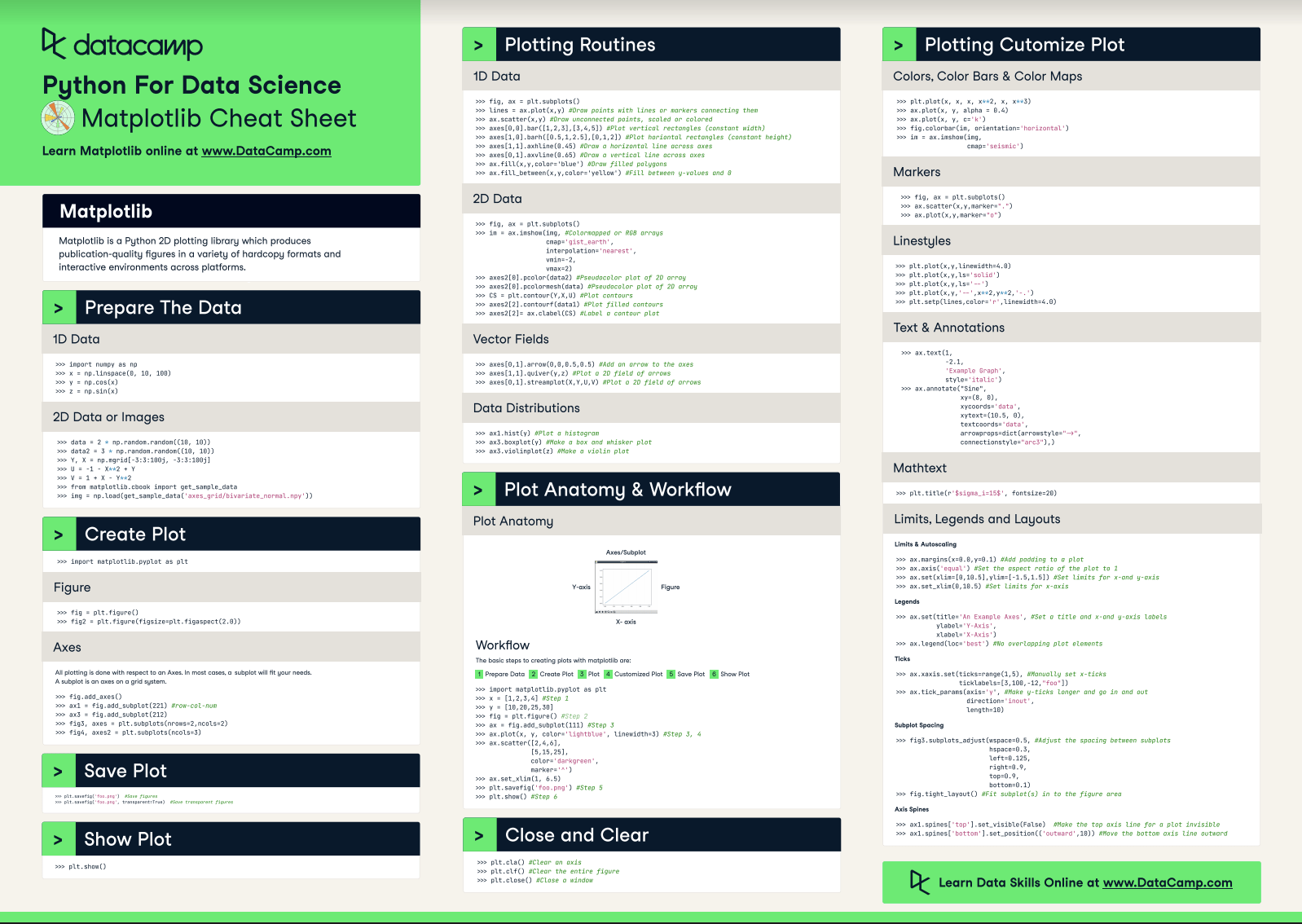With this handy reference, you'll familiarize yourself in no time with the basics of Matplotlib: you'll learn how you can prepare your data, create a new plot, use some basic plotting routines to your advantage, add customizations to your plots, and save, show and close the plots that you make.

What might have looked difficult before will definitely be more clear once you start using this cheat sheet! Use it in combination with the Matplotlib Gallery, the documentation.

Matplotlib

Matplotlib is a Python 2D plotting library which produces publication-quality figures in a variety of hardcopy formats and interactive environments across platforms.

## Prepare the Data

### 1D Data

``````>>> import numpy as np
>>> x = np.linspace(0, 10, 100)
>>> y = np.cos(x)
>>> z = np.sin(x)``````

### 2D Data or Images

``````>>> data = 2 * np.random.random((10, 10))
>>> data2 = 3 * np.random.random((10, 10))
>>> Y, X = np.mgrid[-3:3:100j, -3:3:100j]
>>> U = 1 X** 2 + Y
>>> V = 1 + X Y**2
>>> from matplotlib.cbook import get_sample_data

## Create Plot

``>>> import matplotlib.pyplot as plt``

### Figure

``````>>> fig = plt.figure()
>>> fig2 = plt.figure(figsize=plt.figaspect(2.0))``````

### Axes

``````>>> fig.add_axes()
>>> fig3, axes = plt.subplots(nrows=2,ncols=2)
>>> fig4, axes2 = plt.subplots(ncols=3)``````

## Save Plot

``````>>> plt.savefig('foo.png') #Save figures
>>> plt.savefig('foo.png',  transparent=True) #Save transparent figures``````

## Show Plot

``>>> plt.show()``

## 1D Data

``````>>> fig, ax = plt.subplots()
>>> lines = ax.plot(x,y) #Draw points with lines or markers connecting them
>>> ax.scatter(x,y) #Draw unconnected points, scaled or colored
>>> axes[0,0].bar([1,2,3],[3,4,5]) #Plot vertical rectangles (constant width)
>>> axes[1,0].barh([0.5,1,2.5],[0,1,2]) #Plot horiontal rectangles (constant height)
>>> axes[1,1].axhline(0.45) #Draw a horizontal line across axes
>>> axes[0,1].axvline(0.65) #Draw a vertical line across axes
>>> ax.fill(x,y,color='blue') #Draw filled polygons
>>> ax.fill_between(x,y,color='yellow') #Fill between y values and 0``````

### 2D Data

``````>>> fig, ax = plt.subplots()
>>> im = ax.imshow(img, #Colormapped or RGB arrays
cmap= 'gist_earth',
interpolation= 'nearest',
vmin=-2,
vmax=2)
>>> axes2.pcolor(data2) #Pseudocolor plot of 2D array
>>> axes2.pcolormesh(data) #Pseudocolor plot of 2D array
>>> CS = plt.contour(Y,X,U) #Plot contours
>>> axes2.contourf(data1) #Plot filled contours
>>> axes2= ax.clabel(CS) #Label a contour plot``````

### Vector Fields

``````>>> axes[0,1].arrow(0,0,0.5,0.5) #Add an arrow to the axes
>>> axes[1,1].quiver(y,z) #Plot a 2D field of arrows
>>> axes[0,1].streamplot(X,Y,U,V) #Plot a 2D field of arrows``````

### Data Distributions

``````>>> ax1.hist(y) #Plot a histogram
>>> ax3.boxplot(y) #Make a box and whisker plot
>>> ax3.violinplot(z)  #Make a violin plot``````

## Plot Anatomy & Workflow

### Plot Anatomy

y-axis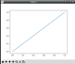x-axis

### Workflow

The basic steps to creating plots with matplotlib are:

1 Prepare Data
2 Create Plot
3 Plot
4 Customized Plot
5 Save Plot
6 Show Plot

``````>>> import matplotlib.pyplot as plt
>>> x = [1,2,3,4]  #Step 1
>>> y = [10,20,25,30]
>>> fig = plt.figure() #Step 2
>>> ax = fig.add_subplot(111) #Step 3
>>> ax.plot(x, y, color= 'lightblue', linewidth=3)  #Step 3, 4
>>> ax.scatter([2,4,6],
[5,15,25],
color= 'darkgreen',
marker= '^' )
>>> ax.set_xlim(1, 6.5)
>>> plt.savefig('foo.png' ) #Step 5
>>> plt.show() #Step 6``````

## Close and Clear

``````>>> plt.cla()  #Clear an axis
>>> plt.clf(). #Clear the entire figure
>>> plt.close(). #Close a window``````

## Plotting Customize Plot

### Colors, Color Bars & Color Maps

``````>>> plt.plot(x, x, x, x**2, x, x** 3)
>>> ax.plot(x, y, alpha = 0.4)
>>> ax.plot(x, y, c= 'k')
>>> fig.colorbar(im, orientation= 'horizontal')
>>> im = ax.imshow(img,
cmap= 'seismic' )``````

### Markers

``````>>> fig, ax = plt.subplots()
>>> ax.scatter(x,y,marker= ".")
>>> ax.plot(x,y,marker= "o")``````

### Linestyles

``````>>> plt.plot(x,y,linewidth=4.0)
>>> plt.plot(x,y,ls= 'solid')
>>> plt.plot(x,y,ls= '--')
>>> plt.plot(x,y,'--' ,x**2,y**2,'-.' )
>>> plt.setp(lines,color= 'r',linewidth=4.0)``````

### Text & Annotations

``````>>> ax.text(1,
-2.1,
'Example Graph',
style= 'italic' )
>>> ax.annotate("Sine",
xy=(8, 0),
xycoords= 'data',
xytext=(10.5, 0),
textcoords= 'data',
arrowprops=dict(arrowstyle= "->",
connectionstyle="arc3"),)``````

### Mathtext

``>>> plt.title(r '\$sigma_i=15\$', fontsize=20)``

### Limits, Legends and Layouts

Limits & Autoscaling

``````>>> ax.margins(x=0.0,y=0.1) #Add padding to a plot
>>> ax.axis('equal')  #Set the aspect ratio of the plot to 1
>>> ax.set(xlim=[0,10.5],ylim=[-1.5,1.5])  #Set limits for x-and y-axis
>>> ax.set_xlim(0,10.5) #Set limits for x-axis``````

Legends

``````>>> ax.set(title= 'An Example Axes',  #Set a title and x-and y-axis labels
ylabel= 'Y-Axis',
xlabel= 'X-Axis')
>>> ax.legend(loc= 'best')  #No overlapping plot elements``````

Ticks

``````>>> ax.xaxis.set(ticks=range(1,5),  #Manually set x-ticks
ticklabels=[3,100, 12,"foo" ])
>>> ax.tick_params(axis= 'y', #Make y-ticks longer and go in and out
direction= 'inout',
length=10)``````

Subplot Spacing

``````>>> fig3.subplots_adjust(wspace=0.5,   #Adjust the spacing between subplots
hspace=0.3,
left=0.125,
right=0.9,
top=0.9,
bottom=0.1)
>>> fig.tight_layout() #Fit subplot(s) in to the figure area``````

Axis Spines

``````>>> ax1.spines[ 'top'].set_visible(False) #Make the top axis line for a plot invisible
>>> ax1.spines['bottom' ].set_position(( 'outward',10))  #Move the bottom axis line outward``````

Have this Cheat Sheet at your fingertips

Original article source at https://www.datacamp.com

#matplotlib #cheatsheet #python1653464648

## Python Data Visualization: Bokeh Cheat Sheet

A handy cheat sheet for interactive plotting and statistical charts with Bokeh.

Bokeh distinguishes itself from other Python visualization libraries such as Matplotlib or Seaborn in the fact that it is an interactive visualization library that is ideal for anyone who would like to quickly and easily create interactive plots, dashboards, and data applications.

Bokeh is also known for enabling high-performance visual presentation of large data sets in modern web browsers.

For data scientists, Bokeh is the ideal tool to build statistical charts quickly and easily; But there are also other advantages, such as the various output options and the fact that you can embed your visualizations in applications. And let's not forget that the wide variety of visualization customization options makes this Python library an indispensable tool for your data science toolbox.

Now, DataCamp has created a Bokeh cheat sheet for those who have already taken the course and that still want a handy one-page reference or for those who need an extra push to get started.

In short, you'll see that this cheat sheet not only presents you with the five steps that you can go through to make beautiful plots but will also introduce you to the basics of statistical charts.

In no time, this Bokeh cheat sheet will make you familiar with how you can prepare your data, create a new plot, add renderers for your data with custom visualizations, output your plot and save or show it. And the creation of basic statistical charts will hold no secrets for you any longer.

Boost your Python data visualizations now with the help of Bokeh! :)

## Plotting With Bokeh

The Python interactive visualization library Bokeh enables high-performance visual presentation of large datasets in modern web browsers.

Bokeh's mid-level general-purpose bokeh. plotting interface is centered around two main components: data and glyphs.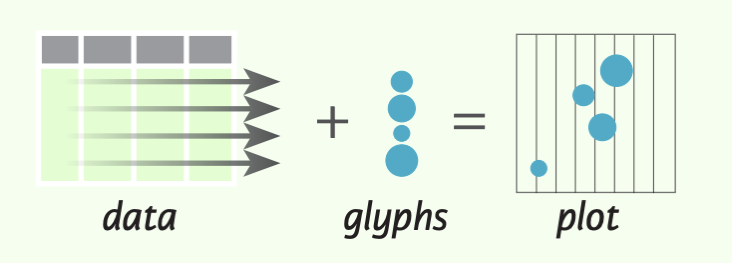The basic steps to creating plots with the bokeh. plotting interface are:

1. Prepare some data (Python lists, NumPy arrays, Pandas DataFrames and other sequences of values)
2. Create a new plot
4. Specify where to generate the output
5. Show or save the results
``````>>> from bokeh.plotting import figure
>>> from bokeh.io import output_file, show
>>> x = [1, 2, 3, 4, 5] #Step 1
>>> y = [6, 7, 2, 4, 5]
>>> p = figure(title="simple line example", #Step 2
x_axis_label='x',
y_axis_label='y')
>>> p.line(x, y, legend="Temp.", line_width=2) #Step 3
>>> output_file("lines.html") #Step 4
>>> show(p) #Step 5``````

## 1. Data

Under the hood, your data is converted to Column Data Sources. You can also do this manually:

``````>>> import numpy as np
>>> import pandas as pd
>>> df = pd.OataFrame(np.array([[33.9,4,65, 'US'], [32.4, 4, 66, 'Asia'], [21.4, 4, 109, 'Europe']]),
columns= ['mpg', 'cyl',   'hp',   'origin'],
index=['Toyota', 'Fiat', 'Volvo'])

>>> from bokeh.models import ColumnOataSource
>>> cds_df = ColumnOataSource(df)``````

## 2. Plotting

``````>>> from bokeh.plotting import figure
>>>p1= figure(plot_width=300, tools='pan,box_zoom')
>>> p2 = figure(plot_width=300, plot_height=300,
x_range=(0, 8), y_range=(0, 8))
>>> p3 = figure()``````

## 3. Renderers & Visual Customizations

### Glyphs

Scatter Markers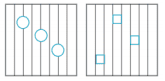``````>>> p1.circle(np.array([1,2,3]), np.array([3,2,1]), fill_color='white')
>>> p2.square(np.array([1.5,3.5,5.5]), [1,4,3],
color='blue', size=1)
``````

Line Glyphs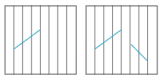``````>>> pl.line([1,2,3,4], [3,4,5,6], line_width=2)
>>> p2.multi_line(pd.DataFrame([[1,2,3],[5,6,7]]),
pd.DataFrame([[3,4,5],[3,2,1]]),
color="blue")
``````

### Customized Glyphs

Selection and Non-Selection Glyphs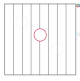``````>>> p = figure(tools='box_select')
>>> p. circle ('mpg', 'cyl', source=cds_df,
selection_color='red',
nonselection_alpha=0.1)
``````

Hover Glyphs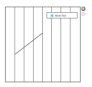``````>>> from bokeh.models import HoverTool
>>>hover= HoverTool(tooltips=None, mode='vline')
``````

Color Mapping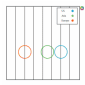``````>>> from bokeh.models import CategoricalColorMapper
>>> color_mapper = CategoricalColorMapper(
factors= ['US', 'Asia', 'Europe'],
palette= ['blue', 'red', 'green'])
>>>  p3. circle ('mpg', 'cyl', source=cds_df,
color=dict(field='origin',
transform=color_mapper), legend='Origin')
``````

## 4. Output & Export

### Notebook

``````>>> from bokeh.io import output_notebook, show
>>> output_notebook()
``````

### HTML

Standalone HTML

``````>>> from bokeh.embed import file_html
>>> from bokeh.resources import CON
>>> html = file_html(p, CON, "my_plot")

>>> from  bokeh.io  import  output_file,  show
>>> output_file('my_bar_chart.html',  mode='cdn')``````

Components

``````>>> from bokeh.embed import components
>>> script, div= components(p)``````

### PNG

``````>>> from bokeh.io import export_png
>>> export_png(p, filename="plot.png")``````

### SVG

``````>>> from bokeh.io import export_svgs
>>> p. output_backend = "svg"
>>> export_svgs(p,filename="plot.svg")``````

### Legend Location

Inside Plot Area

``````>>> p.legend.location = 'bottom left'
``````

Outside Plot Area

``````>>> from bokeh.models import Legend
>>> r1 = p2.asterisk(np.array([1,2,3]), np.array([3,2,1])
>>> r2 = p2.line([1,2,3,4], [3,4,5,6])
>>> legend = Legend(items=[("One" ,[p1, r1]),("Two",[r2])], location=(0, -30))

### Legend Background & Border

``````>>> p.legend. border_line_color = "navy"
>>> p.legend.background_fill_color = "white"``````

### Legend Orientation

``````>>> p.legend.orientation = "horizontal"
>>> p.legend.orientation = "vertical"``````

### Rows & Columns Layout

Rows

``````>>> from bokeh.layouts import row
>>>layout= row(p1,p2,p3)
``````

Columns

``````>>> from bokeh.layouts import columns
>>>layout= column(p1,p2,p3)``````

Nesting Rows & Columns

``````>>>layout= row(column(p1,p2), p3)
``````

### Grid Layout

``````>>> from bokeh.layouts import gridplot
>>> rowl = [p1,p2]
>>> row2 = [p3]
>>> layout = gridplot([[p1, p2],[p3]])``````

### Tabbed Layout

``````>>> from bokeh.models.widgets import Panel, Tabs
>>> tab1 = Panel(child=p1, title="tab1")
>>> tab2 = Panel(child=p2, title="tab2")
>>> layout = Tabs(tabs=[tab1, tab2])``````

``````Linked Axes
>>> p2.x_range = p1.x_range
>>> p2.y_range = p1.y_range``````

``````>>> p4 = figure(plot_width = 100, tools='box_select,lasso_select')
>>> p4.circle('mpg', 'cyl' , source=cds_df)
>>> p5 = figure(plot_width = 200, tools='box_select,lasso_select')
>>> p5.circle('mpg', 'hp', source=cds df)
>>>layout= row(p4,p5)``````

## 5. Show or Save Your Plots

``````>>> show(p1)
>>> show(layout)
>>> save(p1)``````

Have this Cheat Sheet at your fingertips

Original article source at https://www.datacamp.com

#python #datavisualization #bokeh #cheatsheet1641805837

## How to Predict Housing Prices with Linear Regression?The final objective is to estimate the cost of a certain house in a Boston suburb. In 1970, the Boston Standard Metropolitan Statistical Area provided the information. To examine and modify the data, we will use several techniques such as data pre-processing and feature engineering. After that, we'll apply a statistical model like regression model to anticipate and monitor the real estate market.

Project Outline:

• EDA
• Feature Engineering
• Pick and Train a Model
• Interpret
• Conclusion

### EDA

Before using a statistical model, the EDA is a good step to go through in order to:

• Recognize the data set
• Check to see if any information is missing.
• Find some outliers.
• To get more out of the data, add, alter, or eliminate some features.

### Importing the Libraries

• Recognize the data set
• Check to see if any information is missing.
• Find some outliers.
• To get more out of the data, add, alter, or eliminate some features.

# Import the libraries #Dataframe/Numerical libraries import pandas as pd import numpy as np #Data visualization import plotly.express as px import matplotlib import matplotlib.pyplot as plt import seaborn as sns #Machine learning model from sklearn.linear_model import LinearRegression

### Have a Look at the Columns

Crime: It refers to a town's per capita crime rate.

ZN: It is the percentage of residential land allocated for 25,000 square feet.

Indus: The amount of non-retail business lands per town is referred to as the indus.

CHAS: CHAS denotes whether or not the land is surrounded by a river.

NOX: The NOX stands for nitric oxide content (part per 10m)

RM: The average number of rooms per home is referred to as RM.

AGE: The percentage of owner-occupied housing built before 1940 is referred to as AGE.

DIS: Weighted distance to five Boston employment centers are referred to as dis.

TAX: The TAX columns denote the rate of full-value property taxes per \$10,000 dollars.

B: B=1000(Bk — 0.63)2 is the outcome of the equation, where Bk is the proportion of blacks in each town.

PTRATIO: It refers to the student-to-teacher ratio in each community.

LSTAT: It refers to the population's lower socioeconomic status.

MEDV: It refers to the 1000-dollar median value of owner-occupied residences.

### Data Preprocessing

# Check if there is any missing values. housing_df.isna().sum() CRIM       0 ZN         0 INDUS      0 CHAS       0 NOX        0 RM         0 AGE        0 DIS        0 RAD        0 TAX        0 PTRATIO    0 B          0 LSTAT      0 MEDV       0 dtype: int64

No missing values are found

We examine our data's mean, standard deviation, and percentiles.

housing_df.describe()The crime, area, sector, nitric oxides, 'B' appear to have multiple outliers at first look because the minimum and maximum values are so far apart. In the Age columns, the mean and the Q2(50 percentile) do not match.

We might double-check it by examining the distribution of each column.

### Inferences

1. The rate of crime is rather low. The majority of values are in the range of 0 to 25. With a huge value and a value of zero.
2. The majority of residential land is zoned for less than 25,000 square feet. Land zones larger than 25,000 square feet represent a small portion of the dataset.
3. The percentage of non-retial commercial acres is mostly split between two ranges: 0-13 and 13-23.
4. The majority of the properties are bordered by the river, although a tiny portion of the data is not.
5. The content of nitrite dioxide has been trending lower from.3 to.7, with a little bump towards.8. It is permissible to leave a value in the range of 0.1–1.
6. The number of rooms tends to cluster around the average.
7. With time, the proportion of owner-occupied units rises.
8. As the number of weights grows, the weight distance between 5 employment centers reduces. It could indicate that individuals choose to live in new high-employment areas.
9. People choose to live in places with limited access to roadways (0-10). We have a 30th percentile outlier.
10. The majority of dwelling taxes are in the range of \$200-450, with large outliers around \$700,000.
11. The percentage of people with lower status tends to cluster around the median. The majority of persons are of lower social standing.

Because the model is overly generic, removing all outliers will underfit it. Keeping all outliers causes the model to overfit and become excessively accurate. The data's noise will be learned.

The approach is to establish a happy medium that prevents the model from becoming overly precise. When faced with a new set of data, however, they generalise well.

We'll keep numbers below 600 because there's a huge anomaly in the TAX column around 600.

new_df=housing_df[housing_df['TAX']<600]

### Looking at the DistributionThe overall distribution, particularly the TAX, PTRATIO, and RAD, has improved slightly.

### CorrelationPerfect correlation is denoted by the clear values. The medium correlation between the columns is represented by the reds, while the negative correlation is represented by the black.

With a value of 0.89, we can see that 'MEDV', which is the medium price we wish to anticipate, is substantially connected with the number of rooms 'RM'. The proportion of black people in area 'B' with a value of 0.19 is followed by the residential land 'ZN' with a value of 0.32 and the percentage of black people in area 'ZN' with a value of 0.32.

The metrics that are most connected with price will be plotted.## Feature Engineering

### Feature Scaling

Gradient descent is aided by feature scaling, which ensures that all features are on the same scale. It makes locating the local optimum much easier.

Mean standardization is one strategy to employ. It substitutes (target-mean) for the target to ensure that the feature has a mean of nearly zero.

def standard(X):    '''Standard makes the feature 'X' have a zero mean'''    mu=np.mean(X) #mean    std=np.std(X) #standard deviation    sta=(X-mu)/std # mean normalization    return mu,std,sta     mu,std,sta=standard(X) X=sta X

### Choose and Train the Model

For the sake of the project, we'll apply linear regression.

Typically, we run numerous models and select the best one based on a particular criterion.

Linear regression is a sort of supervised learning model in which the response is continuous, as it relates to machine learning.

Form of Linear Regression

y= θX+θ1 or y= θ1+X1θ2 +X2θ3 + X3θ4

y is the target you will be predicting

0 is the coefficient

x is the input

We will Sklearn to develop and train the model

#Import the libraries to train the model from sklearn.model_selection import train_test_split from sklearn.linear_model import LinearRegression

Allow us to utilise the train/test method to learn a part of the data on one set and predict using another set using the train/test approach.

X_train,X_test,y_train,y_test=train_test_split(X,y,test_size=0.4) #Create and Train the model model=LinearRegression().fit(X_train,y_train) #Generate prediction predictions_test=model.predict(X_test) #Compute loss to evaluate the model coefficient= model.coef_ intercept=model.intercept_ print(coefficient,intercept) [7.22218258] 24.66379606613584

In this example, you will learn the model using below hypothesis:

Price= 24.85 + 7.18* Room

It is interpreted as:

For a decided price of a house:

A 7.18-unit increase in the price is connected with a growth in the number of rooms.

As a side note, this is an association, not a cause!

### Interpretation

You will need a metric to determine whether our hypothesis was right. The RMSE approach will be used.

Root Means Square Error (RMSE) is defined as the square root of the mean of square error. The difference between the true and anticipated numbers called the error. It's popular because it can be expressed in y-units, which is the median price of a home in our scenario.

def rmse(predict,actual):    return np.sqrt(np.mean(np.square(predict - actual))) # Split the Data into train and test set X_train,X_test,y_train,y_test=train_test_split(X,y,test_size=0.4) #Create and Train the model model=LinearRegression().fit(X_train,y_train) #Generate prediction predictions_test=model.predict(X_test) #Compute loss to evaluate the model coefficient= model.coef_ intercept=model.intercept_ print(coefficient,intercept) loss=rmse(predictions_test,y_test) print('loss: ',loss) print(model.score(X_test,y_test)) #accuracy [7.43327725] 24.912055881970886 loss: 3.9673165450580714 0.7552661033654667 Loss will be 3.96

This means that y-units refer to the median value of occupied homes with 1000 dollars.

This will be less by 3960 dollars.

While learning the model you will have a high variance when you divide the data. Coefficient and intercept will vary. It's because when we utilized the train/test approach, we choose a set of data at random to place in either the train or test set. As a result, our theory will change each time the dataset is divided.

This problem can be solved using a technique called cross-validation.

### Improvisation in the Model

With 'Forward Selection,' we'll iterate through each parameter to assist us choose the numbers characteristics to include in our model.

### Forward Selection

1. Choose the most appropriate variable (in our case based on high correlation)
2. Add the next best variable to the model
3. Some predetermined conditions must meet.

We'll use a random state of 1 so that each iteration yields the same outcome.

cols=[] los=[] los_train=[] scor=[] i=0 while i < len(high_corr_var):    cols.append(high_corr_var[i])        # Select inputs variables    X=new_df[cols]        #mean normalization    mu,std,sta=standard(X)    X=sta        # Split the data into training and testing    X_train,X_test,y_train,y_test= train_test_split(X,y,random_state=1)        #fit the model to the training    lnreg=LinearRegression().fit(X_train,y_train)        #make prediction on the training test    prediction_train=lnreg.predict(X_train)        #make prediction on the testing test    prediction=lnreg.predict(X_test)        #compute the loss on train test    loss=rmse(prediction,y_test)    loss_train=rmse(prediction_train,y_train)    los_train.append(loss_train)    los.append(loss)        #compute the score    score=lnreg.score(X_test,y_test)    scor.append(score)        i+=1

We have a big 'loss' with a smaller collection of variables, yet our system will overgeneralize in this scenario. Although we have a reduced 'loss,' we have a large number of variables. However, if the model grows too precise, it may not generalize well to new data.

In order for our model to generalize well with another set of data, we might use 6 or 7 features. The characteristic chosen is descending based on how strong the price correlation is.

high_corr_var ['RM', 'ZN', 'B', 'CHAS', 'RAD', 'DIS', 'CRIM', 'NOX', 'AGE', 'TAX', 'INDUS', 'PTRATIO', 'LSTAT']

With 'RM' having a high price correlation and LSTAT having a negative price correlation.

# Create a list of features names feature_cols=['RM','ZN','B','CHAS','RAD','CRIM','DIS','NOX'] #Select inputs variables X=new_df[feature_cols] # Split the data into training and testing sets X_train,X_test,y_train,y_test= train_test_split(X,y, random_state=1) # feature engineering mu,std,sta=standard(X) X=sta # fit the model to the trainning data lnreg=LinearRegression().fit(X_train,y_train) # make prediction on the testing test prediction=lnreg.predict(X_test) # compute the loss loss=rmse(prediction,y_test) print('loss: ',loss) lnreg.score(X_test,y_test) loss: 3.212659865936143 0.8582338376696363

The test set yielded a loss of 3.21 and an accuracy of 85%.

Other factors, such as alpha, the learning rate at which our model learns, could still be tweaked to improve our model. Alternatively, return to the preprocessing section and working to increase the parameter distribution.

For more details regarding scraping real estate data you can contact Scraping Intelligence today

https://www.websitescraper.com/how-to-predict-housing-prices-with-linear-regression.php